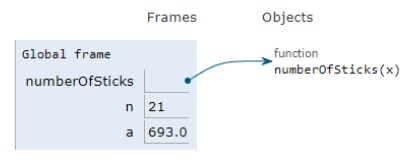# Python Program for Triangular Matchstick Number

PythonServer Side ProgrammingProgramming

Problem statement − We are given a number X which represents the floor of a matchstick pyramid, we need to display the total number of matchstick required to form a pyramid of matchsticks with x floors.

Now let’s observe the solution in the implementation below −

## Example

Live Demo

#function
def numberOfSticks(x):
return (3 * x * (x + 1)) / 2
# main()
n=21
a=numberOfSticks(n)
print(int(a))

## Output

693All the variables are declared in the local scope and their references are seen in the figure above.

## Conclusion

In this article, we have learned about how we can make a Python Program for Triangular Matchstick Number